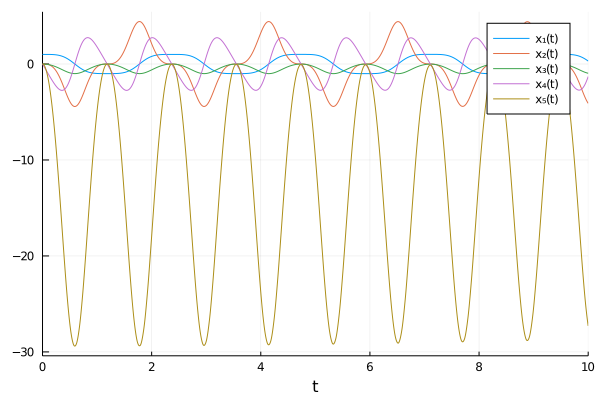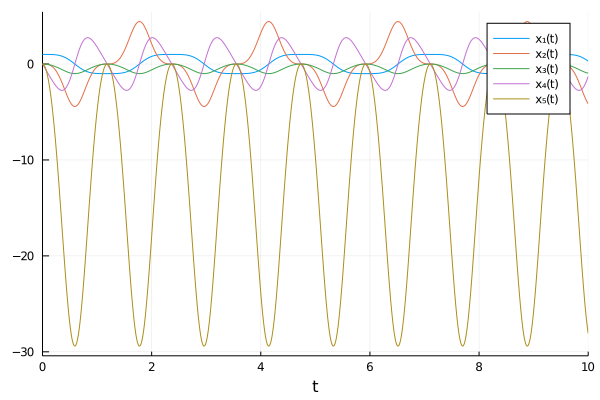# Automated Index Reduction of DAEs

In many cases one may accidentally write down a DAE that is not easily solvable by numerical methods. In this tutorial we will walk through an example of a pendulum which accidentally generates an index-3 DAE, and show how to use the modelingtoolkitize to correct the model definition before solving.

## Copy-Pastable Example

using ModelingToolkit
using LinearAlgebra
using OrdinaryDiffEq
using Plots

function pendulum!(du, u, p, t)
x, dx, y, dy, T = u
g, L = p
du = dx
du = T*x
du = dy
du = T*y - g
du = x^2 + y^2 - L^2
return nothing
end
pendulum_fun! = ODEFunction(pendulum!, mass_matrix=Diagonal([1,1,1,1,0]))
u0 = [1.0, 0, 0, 0, 0]
p = [9.8, 1]
tspan = (0, 10.0)
pendulum_prob = ODEProblem(pendulum_fun!, u0, tspan, p)
traced_sys = modelingtoolkitize(pendulum_prob)
pendulum_sys = structural_simplify(traced_sys)
prob = ODAEProblem(pendulum_sys, Pair[], tspan)
sol = solve(prob, Tsit5(),abstol=1e-8,reltol=1e-8)
plot(sol, vars=states(traced_sys))

## Explanation

### Attempting to Solve the Equation

In this tutorial we will look at the pendulum system:

\begin{aligned} x^\prime &= v_x\\ v_x^\prime &= Tx\\ y^\prime &= v_y\\ v_y^\prime &= Ty - g\\ 0 &= x^2 + y^2 - L^2 \end{aligned}

As a good DifferentialEquations.jl user, one would follow the mass matrix DAE tutorial to arrive at code for simulating the model:

using OrdinaryDiffEq, LinearAlgebra
function pendulum!(du, u, p, t)
x, dx, y, dy, T = u
g, L = p
du = dx; du = T*x
du = dy; du = T*y - g
du = x^2 + y^2 - L^2
end
pendulum_fun! = ODEFunction(pendulum!, mass_matrix=Diagonal([1,1,1,1,0]))
u0 = [1.0, 0, 0, 0, 0]; p = [9.8, 1]; tspan = (0, 10.0)
pendulum_prob = ODEProblem(pendulum_fun!, u0, tspan, p)
solve(pendulum_prob,Rodas4())

However, one will quickly be greeted with the unfortunate message:

┌ Warning: First function call produced NaNs. Exiting.
└ @ OrdinaryDiffEq C:\Users\accou\.julia\packages\OrdinaryDiffEq\yCczp\src\initdt.jl:76
┌ Warning: Automatic dt set the starting dt as NaN, causing instability.
└ @ OrdinaryDiffEq C:\Users\accou\.julia\packages\OrdinaryDiffEq\yCczp\src\solve.jl:485
┌ Warning: NaN dt detected. Likely a NaN value in the state, parameters, or derivative value caused this outcome.
└ @ SciMLBase C:\Users\accou\.julia\packages\SciMLBase\DrPil\src\integrator_interface.jl:325

Did you implement the DAE incorrectly? No. Is the solver broken? No.

### Understanding DAE Index

It turns out that this is a property of the DAE that we are attempting to solve. This kind of DAE is known as an index-3 DAE. For a complete discussion of DAE index, see this article. Essentially the issue here is that we have 4 differential variables ($x$, $v_x$, $y$, $v_y$) and one algebraic variable $T$ (which we can know because there is no D(T) term in the equations). An index-1 DAE always satisfies that the Jacobian of the algebraic equations is non-singular. Here, the first 4 equations are differential equations, with the last term the algebraic relationship. However, the partial derivative of x^2 + y^2 - L^2 w.r.t. T is zero, and thus the Jacobian of the algebraic equations is the zero matrix and thus it's singular. This is a very quick way to see whether the DAE is index 1!

The problem with higher order DAEs is that the matrices used in Newton solves are singular or close to singular when applied to such problems. Because of this fact, the nonlinear solvers (or Rosenbrock methods) break down, making them difficult to solve. The classic paper DAEs are not ODEs goes into detail on this and shows that many methods are no longer convergent when index is higher than one. So it's not necessarily the fault of the solver or the implementation: this is known.

But that's not a satisfying answer, so what do you do about it?

### Transforming Higher Order DAEs to Index-1 DAEs

It turns out that higher order DAEs can be transformed into lower order DAEs. If you differentiate the last equation two times and perform a substitution, you can arrive at the following set of equations:

\begin{aligned} x^\prime =& v_x \\ v_x^\prime =& x T \\ y^\prime =& v_y \\ v_y^\prime =& y T - g \\ 0 =& 2 \left(v_x^{2} + v_y^{2} + y ( y T - g ) + T x^2 \right) \end{aligned}

Note that this is mathematically-equivalent to the equation that we had before, but the Jacobian w.r.t. T of the algebraic equation is no longer zero because of the substitution. This means that if you wrote down this version of the model it will be index-1 and solve correctly! In fact, this is how DAE index is commonly defined: the number of differentiations it takes to transform the DAE into an ODE, where an ODE is an index-0 DAE by substituting out all of the algebraic relationships.

### Automating the Index Reduction

However, requiring the user to sit there and work through this process on potentially millions of equations is an unfathomable mental overhead. But, we can avoid this by using methods like the Pantelides algorithm for automatically performing this reduction to index 1. While this requires the ModelingToolkit symbolic form, we use modelingtoolkitize to transform the numerical code into symbolic code, run dae_index_lowering lowering, then transform back to numerical code with ODEProblem, and solve with a numerical solver. Let's try that out:

traced_sys = modelingtoolkitize(pendulum_prob)
pendulum_sys = structural_simplify(dae_index_lowering(traced_sys))
prob = ODEProblem(pendulum_sys, Pair[], tspan)
sol = solve(prob, Rodas4())

using Plots
plot(sol, vars=states(traced_sys))Note that plotting using states(traced_sys) is done so that any variables which are symbolically eliminated, or any variable reorderings done for enhanced parallelism/performance, still show up in the resulting plot and the plot is shown in the same order as the original numerical code.

Note that we can even go a little bit further. If we use the ODAEProblem constructor, we can remove the algebraic equations from the states of the system and fully transform the index-3 DAE into an index-0 ODE which can be solved via an explicit Runge-Kutta method:

traced_sys = modelingtoolkitize(pendulum_prob)
pendulum_sys = structural_simplify(dae_index_lowering(traced_sys))
prob = ODAEProblem(pendulum_sys, Pair[], tspan)
sol = solve(prob, Tsit5(),abstol=1e-8,reltol=1e-8)
plot(sol, vars=states(traced_sys))And there you go: this has transformed the model from being too hard to solve with implicit DAE solvers, to something that is easily solved with explicit Runge-Kutta methods for non-stiff equations.